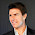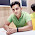# C Program to check Armstrong Number

#### Armstrong Number:

A number is armstrong if the sum of cubes of individual digits of a number is equal to the number itself. For example 371 is an armstrong number as 33 + 73+ 13 = 371. Some other armstrong numbers are: 0, 1, 153, 370, 407.

#### C Program to check Armstrong Number:

```#include <stdio.h>

int main()
{
int n, sum, temp, reminder;
sum = 0;

printf("Enter a number to check Armstrong Number : ");
scanf("%d",&n);

temp = n;
while(temp!=0) {
reminder = temp%10;
sum = sum + reminder*reminder*reminder;
temp = temp / 10;
}
if(n==sum) printf("%d is an Armstrong Number.",n);
else printf("%d is not an Armstrong Number.",n);

return 0;
}
```

#### C Program to  check Armstrong Number using Function

```#include <stdio.h>

int check_arm_num(int x);

int main()
{
int n,sum;

printf("Enter a number to check Armstrong Number : ");
scanf("%d",&n);

sum = check_arm_num(n);

if(n==sum) printf("%d is an Armstrong Number.",n);
else printf("%d is not an Armstrong Number.",n);

return 0;
}

int check_arm_num(int x)
{
int sum = 0, remainder;
while(x!=0){
remainder = x%10;
sum = sum + remainder*remainder*remainder;
x = x / 10;
}
return sum;
}
```
Share:

#### 3 comments :

1.2.Armstrong program in C

3.